If you have a related question, please click the "Ask a related question" button in the top right corner. The newly created question will be automatically linked to this question.

# TIDA-010232: Riso Calculation

Part Number: TIDA-010232
Other Parts Discussed in Thread: AMC3330, TLV6001

Hi Ti,

I use Pspice to simulate reference design TIDA-010232 to calculate RisoP and RisoN, but answers are not correct.

Could you please give me a hand?

Please refer to the schematic and EXCEL below.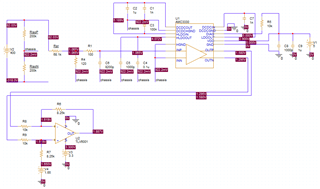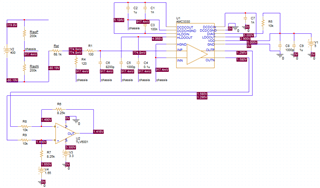• Hello Sky,

Thank for reaching out. There is a mistake in the formulas for calculating RisoN and RisoP.
The correct formulas are:

RisoN = (-(RinAMC + Rst) * (VDC + VN - VP)) / VN

RisoP = ((RinAMC + Rst) * (VDC + VN - VP)) / VP

This is a known problem and the design guide update will be released very soon.

Best regards,

Andreas

• Hi Andreas,

After I fixed my equations, the answers are very close.

• Hi Andreas,

After I fixed my equations, the answers are very close.

• Hi Andreas,

I met a problem about asymmetrical faults calculation.

If I set Riso with different value, the deviation of answers are large.

Is there any solution to solve this problem?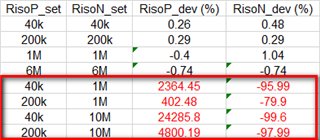• Hi Sky,

For asymmetrical faults the results can be quiet bad for the high value in your cases RisoN. For the lower value in your case RisoP the result accuracy should be still good.

Can you share a screenshot off one of the simulations for asymmetrical faults as you did in the initial post, so I can see where this error is coming from?

Best regards,

Andreas

• Hi Andreas,

I found fomulas mentioned above should be: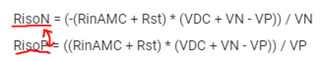Deviations are small with low Riso after I fixed formulas.

Another question, how can I improve deviation with high Riso?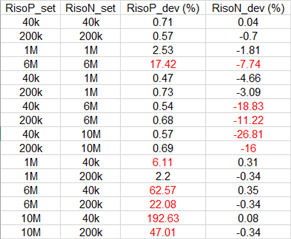• Hi Sky,

Yes you are right, I swapped the the names.

With this approach it is not possible to be very accurate over the complete resistance range, since the ratio between the switched in branch Rst + RinAMC compared to the insulation resistance defines how big the voltage drop at the AMC3330 input is. See the equivalent circuit for SP closed below.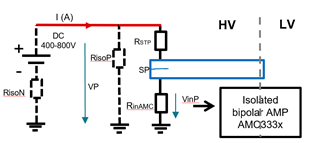If RisoN in this case get very high, VP will be very low and offset errors of AMC3330 and TLV6001 will have a big impact.
Increasing RstP and RinAMC helps in a limited way only since RisoP is parallel to them and limits the resulting equivalent resistance ( RisoP|| (RinAMC+Rst)) which defines VP.

The design was optimized for insulation resistance values between 40k and 200k, since these are typically the critical values in a 400V system. If insulation resistances are high, typically the accuracy is not that important, since low resistance values are the fault scenario.

Best regards,

Andreas